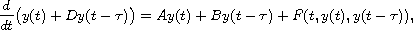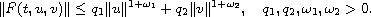Electron. J. Diff. Equ., Vol. 2016 (2016), No. 19, pp. 1-20.

### Exponential stability of solutions to nonlinear time-delay systems of neutral type Gennadii V. Demidenko, Inessa I. Matveeva

Abstract:
We consider a nonlinear time-delay system of neutral equations with constant coefficients in the linear termswhereWe obtain estimates characterizing the exponential decay of solutions at infinity and estimates for attraction sets of the zero solution.

Submitted October 6, 2015. Published January 11, 2016.
Math Subject Classifications: 34K20.
Key Words: Time-delay systems; neutral equation; exponential stability; attraction sets.

Show me the PDF file (298 KB), TEX file for this article.Gennadii V. Demidenko Laboratory of Differential and Difference Equations Sobolev Institute of Mathematics 4, Acad. Koptyug avenue, Novosibirsk 630090, Russia email: demidenk@math.nsc.ru Inessa I. Matveeva Laboratory of Differential and Difference Equations Sobolev Institute of Mathematics 4, Acad. Koptyug avenue, Novosibirsk 630090, Russia email: matveeva@math.nsc.ru